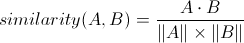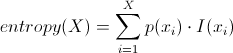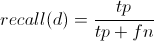## How ProPublica's Message Machine Reverse Engineers Political Microtargeting

How the message machine works.

ProPublica has been collecting political emails for a project we call the Message Machine, with the help of more than 600 readers who have shared demographic information with us and who have so far forwarded us more than 30,000 political emails. The Message Machine identifies and classifies many different emails as different variations of a single email blast, to highlight and analyze the ways in which campaigns use their sizable databases to customize their messages based on what they know about each recipient.

We’ve been publishing our collection for a few months, and today we are publishing the latest results of our analysis. The Machine uses techniques from Natural Language Processing and Machine Learning to analyze every email our readers forward to it.

## TF-IDF

In order to find the variations in language in each email that the Machine receives, the Machine relies on two simple concepts, one called TF-IDF and the other Cosine Similarity.

TF-IDF stands for “Term Frequency-Inverse Document Frequency,” and is a way to assign weights to individual words based on their importance in a collection of documents. It helps us discard common words like ‘the’ and ‘and’ at scale because they should not influence our measure of similarity between documents. TF-IDF is a workhorse of NLP, and it is straightforward to calculate.

First you calculate the Term Frequency for a word:Basically what that says is for every word in a document calculate the number of times it appears, and then divide by the number of times the most common word appears in the document. That represents the term frequency of a particular word. And then you move on to the Inverse Document Frequency:Calculating the Inverse Document Frequency of a word goes like this: take the number of documents you have and divide by the number of documents a given word appears in, and then take the logarithm of the result. Then you multiply the tf and idf together to get tfidf:When you multiply TF and IDF together you get a score representing a value for the importance of the words in a document corrected by their importance in the collection of documents. The effect of this calculation is that rare words are more important than common ones in similarity calculations.

## Cosine Similarity

When the Machine receives an email it needs to find others that have similar content. There are many document similarity algorithms — two that I find most beautiful are the sim hash and min hash algorithms — but I chose a more standard measure called Cosine Similarity. Cosine Similarity is another important technique in NLP and is equally easy to calculate:In concrete terms, Cosine Similarity measures the angle between the two vectors formed by each document’s words (technically, it is the angle between the two hyperplanes that the vectors represent). To calculate Cosine Similarity for two documents you take the dot product of each document’s word vector and divide that by the product of each vector’s magnitude. Because the TF-IDF scores in the documents are all positive, it will produce a number between zero and one. A pair of documents with a cosine similarity of one are identical, and a Cosine Similarity of zero would describe a pair of documents that have no words in common.

Every email blast in the Machine has one or more variations. The machine finds these variations by comparing every new email to the existing variations from each email blast, and if the new email isn’t similar to the existing variations, e.g. below a certain cosine similarity threshold, the Machine creates a new variation.

It is difficult to model these word vectors in a relational database, especially if you want to calculate TF-IDF and Cosine Similarity for the documents quickly. So the Message Machine uses a key-value store database that I built called Daybreak, which we will open source very soon.

A side benefit of storing the word indexes in a custom database is that we can use Cosine Similarity to power a front-facing search engine for the email collection. When a user searches the site, the Machine looks up the query words in our email indexes and returns an list of emails ordered by each email’s Cosine Similarity to the user’s query. The code is really very small.

## Decision Trees and Machine Learning

Once the Machine has clustered the emails and found the variations, it takes the demographics of the users who sent in the email and creates a Machine Learning model called a Decision Tree for every variation of each email &8212; except the most popular one. The Machine throws away the most popular email variation because, after reviewing the data, we found that, by and large, the most popular variation was the one with the least significant variations. In practical terms the most popular variation is likely either the “control” version, or the one sent to folks the campaigns do not know much about.

I decided to use decision trees in the Machine after speaking with Prof. David Karger at MIT. He advised that, like many Machine Learning algorithms, Decision Trees provide results with any amount of data and that the results get better with more examples. More importantly, Decision Trees give easily parsable rules of the classification structure instead of lists of numbers like other Machine Learning classifiers.

Incidentally, Karger is well known for the wonderful algorithm, Karger’s algorithm, that finds the minimum cut of a graph, and you should try to find some time to read up on it.

Decision Trees are useful at finding hidden models in a particular data set, particularly because they produce a human-readable tree of partitions of the data. For example, here is a representation of the Decision Tree model generated for a single Obama for America message:

``````if(donation signal > 69) {
if(state == 21) {
return cluster<19546>
} else {
return cluster<19548>
}
} else {
if(age < 44) {
if(donation signal > 64) {
return cluster<19548>
} else {
return cluster<19546>
}
} else {
return cluster<19545>
}
}
``````

The decision tree algorithm is a very simple tree-building algorithm, in pseudocode:

``````build_tree(data)
return if data.length <= 1
for each attribute in data
calculate information gain on attribute
let @best = maximum information gain
split the data set based on @best into nodes @left and @right
build_tree(@left)
build_tree(@right)
``````

The formula the Machine uses to decide the best split is a straight entropy calculation:Where I is the information content of the value with attribute x at index i:Where P is the probability that the attribute has that value across the data set.

Then the Machine calculates information gain for each split by looping over every possible value for an attribute and by calculating the difference between the entropy of the overall data set and the entropy of splitting the data on that value:Where T denotes the data set for a node. The best split is the one that will produce the highest information gain. The split routine is a binary split function that for categorical variables splits based on whether or not a row’s attribute equals the condition, and for continuous variables, whether or not the attribute is greater or equal to the condition.

After building a tree based on these splits, the Machine prunes the tree by asking each node if it would be better if it were a leaf or if it were a branch by calculating a Laplace Error Estimate for each case. Because the splits may end up with rows that belong to many different email variations, this error estimate throws away decision rules — by converting a branch into a leaf — that were assigned to too many different email variations. Luckily, it is pretty simple to calculate:In the above formula N is the number of examples in a node, k is the number of classes — e.g. email variations — and n is the number of examples belonging to the most popular class.

In order to calculate the accuracy of the models, the Machine holds one example from each email variation back, and then it uses those to test the decision tree's accuracy using a standard calculation called the F Score. An F Score is calculated by counting the true positives (results that returned the right variation), false negatives (results that did not return the right variation) and false positives (results that returned the wrong variation), and then calculating precision, the fraction of returned results that are relevant:and recall, the fraction of relevant instances retrieved:and finally the F Score for the model:Which will return a number between 0 and 1. The machine only assumes that models with an F Score higher than 0.90 are accurate models.

Once the Machine finds an accurate model, it does a Depth First Search of the tree and collects the demographic factors that produced each split. That set of factors is what the Machine presents on individual email pages.

A final note about the demographic information the Machine uses to create the decision trees: the Machine uses basic demographic information like age, sex, household income, state, and whether the user is a likely voter. It also includes an extra bit of information we’re calling a “donation signal” that represents the average previous donation amount asked for by each campaign by user. We infer the "donation signal" from the emails themselves instead of relying on data from our demographic questionnaire. Many Obama for America emails contain a “quick donate” box that asks for more money when a particular constituent has saves his credit card information. If an email has that box we assume the user is a previous donor. Also, some emails explicitly say the recipient is a previous donor.

This extra bit of information is actually the most common predictor of which email a particular person will get, and makes our models much stronger.

If you’d like to see the Message Machine in action, and see how political campaigns are targeting you, please forward political emails you receive to [email protected]

## Latest Stories from ProPublica

### The Mississippi Supreme Court Moved to Ensure Poor Criminal Defendants Would Always Have a Lawyer. It’s Not Working.

Current site Current page